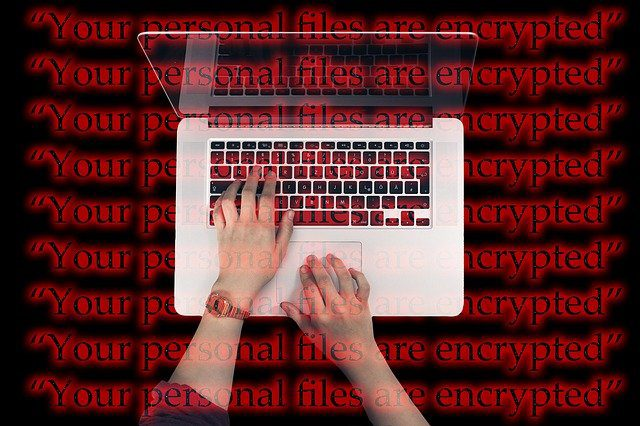# Comprehensive notes Encoding Schemes class 11 cs

Welcome to the study material page for class 11, here I will provide you the Comprehensive notes Encoding Schemes class 11 cs. So let’s begin!

Topics Covered

## Comprehensive notes Encoding Schemes class 11 cs

Encoding Schemes: ASCII, UTF8, UTF32, ISCII, and Unicode are used to represent data into computers. Computers are capable to handle all kinds of data including numbers, text, images, audio, and video files.

As you know that computers do not understand the English alphabets, numbers other than 0 and 1 as well as text symbols. To convert this we use an encoding.Comprehensive notes Encoding Schemes class 11 cs

First of all understand some basic terms related to encoding for Comprehensive notes Encoding Schemes class 11 cs.

## Basic terms related to encoding

1. Encoding Scheme: It is the way or method of conversion in machine language.
2. Code Space: It means all the codes that are used to represent the information. For example, 127 in ASCII code
3. Code Point: It is a code that represents a character in an encoding scheme. For ex. 0x43 represents capital  C letter
4. Code Unit: It refers to the number of bits used in codes.

In the next section of Comprehensive notes Encoding Schemes class 11 cs we will discuss character representations.

## Character / String representation

As you know data is a textual fact, figures, or collection of bytes. So to represent this data into computers we need to convert them into machine language i.e. binary language usually in the form of 0s and 1s.

All the 26 alphabets from A to Z (both upper and lower), numeric digits from 0 to 9 as we are using decimal number systems and other special symbols like @,#,\$,%, etc need to be converted into machine language.

To do this each and every character or digit or special symbol assigned specific code to represent them. These codes are as following:

## ASCII Code

It is a simple code assigned to a character to represent data. ASCII (pronounced as “askee”) is a 7 – bit character code. It represents all the characters available for writing in the file. Every digit in ASCII code represents one byte. Let us have a look at following commonly used characters and code range:

 Characters ASCII Codes A to Z 65 to 90 a to z 97 to 122 0 to 9 48 to 57 Space 32

The computer generally converts these ASCII codes into an equivalent binary number. Let’s understand it by the following example:

Suppose you want to use the capital letter D. So its equivalent ASCII code is 68. This code 68 is a decimal number, ASCII . So the equivalent binary number is 1000100. So finally D = 1000100 for the computers.

There are two versions of ASCII codes:
1. 7 bit: Represents 27 =  128 characters
2. 8 bit: Represents 28 = 256 characters

The next code is ISCII code for Comprehensive notes Encoding Schemes class 11 cs.

## ISCII Code

When computers are used with English language ASCII codes enough to represent data. But as and when the use of computers broadly extended to countries like India, it’s very important to represent data in Indian Languages. So for that in 1991, the Bureau of Indian Standards adopted the Indian Standard Code for Information Interchange has evolved. This code is capable of 8 bits. It is also known as the Indian Scripts Code for Information Interchange. It supports various Indian languages like Devnagri, Gurumukhi, Gujarati, Oriya, Bengali, Assamese, Telugu etc.

The next code for Comprehensive notes Encoding Schemes class 11 cs is Unicode.

## Unicode

Unicode is used for a universal set of characters. As ISCII is used for Indian languages, Unicode is used accepted by the universal standards and represent data in different languages. It was designed for the purpose of representing almost all the languages in computers. The Unicode has different versions like UTF – 8, UTF – 16, UTF – 32.

In this, the numbers in UTF like 8 as 8 bits known as an octet, 16 as 2 – octets, and 32 as 4 – octets representation.

I hope you understood the concept of encoding schemes. For further doubts and queries feel free to ask in the comment section.